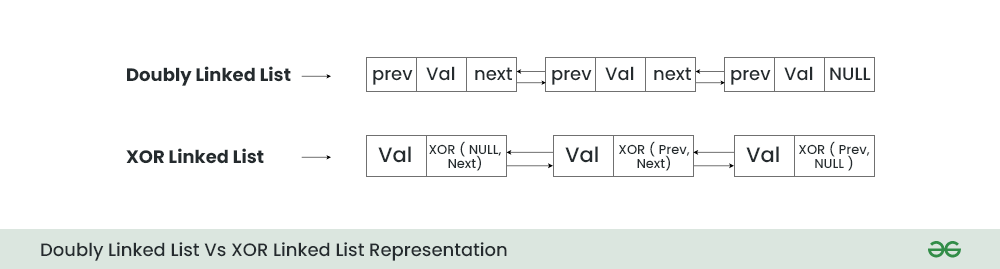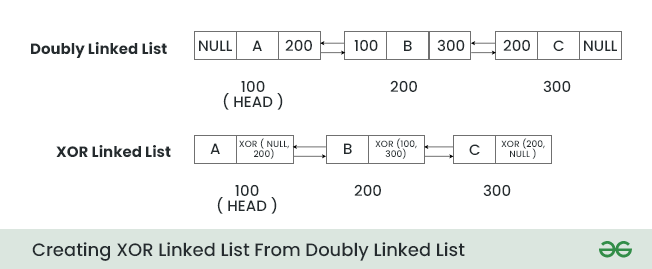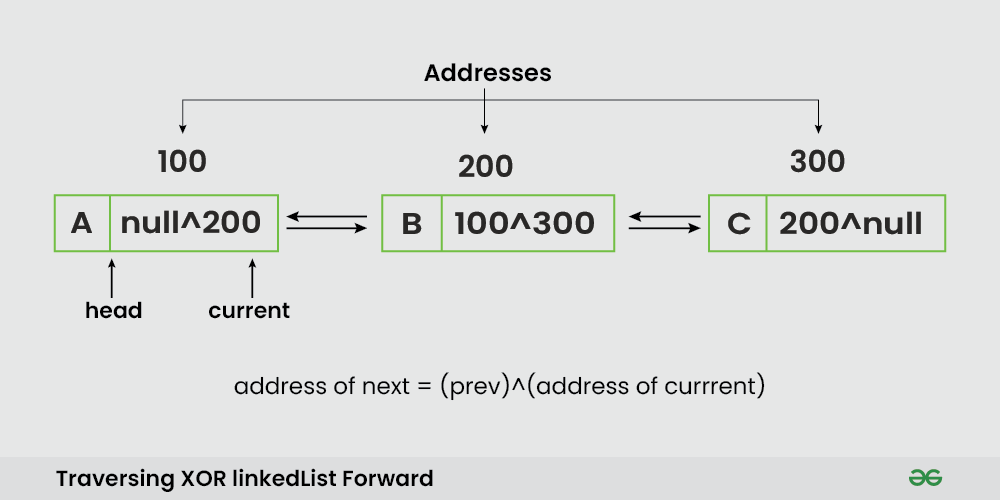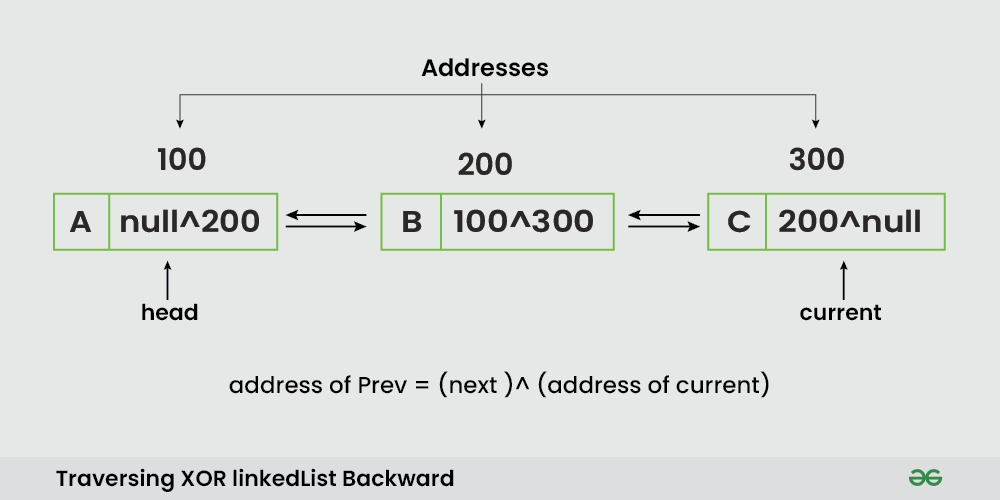# XOR Linked List – A Memory Efficient Doubly Linked List | Set 1

In this post, we’re going to talk about how XOR linked lists are used to reduce the memory requirements of doubly-linked lists.

We know that each node in a doubly-linked list has two pointer fields which contain the addresses of the previous and next node. On the other hand, each node of the XOR linked list requires only a single pointer field, which doesn’t store the actual memory addresses but stores the bitwise XOR of addresses for its previous and next node.Following are the Ordinary and XOR (or Memory Efficient) representations of the Doubly Linked List:In this section, we will discuss both ways in order to demonstrate how XOR representation of doubly linked list differs from ordinary representation of doubly linked list.

1. Ordinary Representation
2. XOR List Representation### Ordinary Representation of doubly linked list.

Node A:
prev = NULL, next = add(B) // previous is NULL and next is address of B

Node B:

Node C:

Node D:
prev = add(C), next = NULL // previous is address of C and next is NULL

### XOR List Representation of doubly linked list.

Lets see the structure of each node of Doubly linked list and XOR linked list:Below is the representation of a node structure for an XOR linked list:

## C++

 `struct` `Node {` `    ``int` `data;`   `    ``// "both": XOR of the previous and next node addresses` `    ``Node* both;` `};`

## Types of XOR Linked List:

There are two main types of XOR Linked List:

1. Singly Linked XOR List: A singly XOR linked list is a variation of the XOR linked list that uses the XOR operation to store the memory address of the next node in a singly linked list. In this type of list, each node stores the XOR of the memory address of the next node and the memory address of the current node.
2. Doubly Linked XOR List: A doubly XOR linked list is a variation of the XOR linked list that uses the XOR operation to store the memory addresses of the next and previous nodes in a doubly linked list. In this type of list, each node stores the XOR of the memory addresses of the next and previous nodes.

## Traversal in XOR linked list:

Two types of traversal are possible in XOR linked list.

1. Forward Traversal
2. Backward Traversal:

### Forward Traversal in XOR linked list:

When traversing the list forward, it’s important to always keep the memory address of the previous element. Address of previous element helps in calculating the address of the next element by the below formula:

Here, “both” is the XOR of address of previous node and address of next node.Forward Traversal of XOR Linked List

Below is the code snippet for forward traversal of the XOR linked list:

## C++

 `Node *prev;` `// Curr points to the first node` `//of the XOR Linked list` `Node *curr = head;` `Node *next;` `While(curr!= NULL)` `{` `  ``cout<data;` `  ``//both represents the XOR value .` `  ``next= prev ^ curr-> both;` `  ``prev= curr;` `  ``curr= next;` `}`

### Backward Traversal in XOR linked list:

When traversing the list backward, it’s important to always keep the memory address of the next element. Address of next element helps in calculating the address of the previous element by the below formula:

Here, “both” is the XOR of address of previous node and address of next node.Backward Traversal of XOR Linked List

Below is the code snippet for backward traversal of the XOR linked list:

## C++

 `// Curr points to the last node` `//of the XOR Linked list` `Node * curr ;` `Node *head;` `Node *prev, *next=NULL;` `while``(curr!=NULL)` `{` `  ``cout<data;` `  ``//both represents the XOR value of the node.` `  ``prev= (next) ^ (curr->both);` `  ``next = curr;` `  ``curr = prev;` `}`

## Basic Operations of XOR Linked list:

• Insertion
• Deletion

### Insertion at Beginning in XOR Linked List:

Below is the steps for insert an element at beginning in XOR Linked List:

• Create a new node , initialize the data and address to the (NULL ^ address of head)
• Then check, If the list is empty, return with that node;

### Insertion at end in XOR Linked List:

Below is the steps for insert an element at end in XOR Linked List:

• Create a new node , initialize the data and address to the (NULL ^ add. of tail)
• Then check, If the list is empty, return with that node;
• Otherwise, assign the XOR of the tail node to the XOR(XOR(tail->both, nullptr), new_node address)

### Deletion at Beginning in XOR Linked List:

Below is the steps for delete an element at beginning in XOR Linked List:

• Check if the head pointer is not null (i.e., the list is not empty).
• Find the next node’s address using XOR by performing XOR(head->both, nullptr)
• Delete the current head node to free up the memory.and Update the head pointer to point to the calculated next node.

### Deletion at End in XOR Linked List:

Below is the steps for delete an element at beginning in XOR Linked List:

• Check if the tail pointer is not null (i.e., the list is not empty).
• If the list is not empty:
• Find the previous node’s address using XOR by performing XOR(tail->both, nullptr). This gives you the previous node in the list.
• Delete the current tail node to free up the memory.
• Update the tail pointer to point to the calculated previous node.

Below is the implementation of the above approach:

## C++

 `// for uintptr_t` `#include ` `#include `   `struct` `Node {` `    ``int` `data;` `    ``// XOR of next and prev` `    ``Node* both;` `};`   `class` `XORLinkedList {` `private``:` `    ``Node* head;` `    ``Node* tail;` `    ``// XOR function for Node pointers` `    ``Node* XOR(Node* a, Node* b);`   `public``:` `    ``// Constructor to initialize an empty` `    ``// list` `    ``XORLinkedList();` `  `  `    ``// Insert a node at the head of the list` `    ``void` `insert_at_head(``int` `data);` `  `  `    ``// Insert a node at the tail of the list` `    ``void` `insert_at_tail(``int` `data);` `  `  `    ``// Delete a node from the head` `    ``// of the list` `    ``void` `delete_from_head();` `  `  `    ``// Delete a node from the tail` `    ``// of the list` `    ``void` `delete_from_tail();` `  `  `    ``// Print the elements of the list` `    ``void` `print_list();` `};`   `XORLinkedList::XORLinkedList()` `{` `    ``head = tail = nullptr; ``// Initialize head and tail to` `                           ``// nullptr for an empty list` `}`   `Node* XORLinkedList::XOR(Node* a, Node* b)` `{` `    ``return` `(` `      `  `        ``// XOR operation for Node pointers` `        ``Node*)((``uintptr_t``)(a) ^ (``uintptr_t``)(b));` `}`   `void` `XORLinkedList::insert_at_head(``int` `data)` `{` `    ``Node* new_node = ``new` `Node();` `    ``new_node->data = data;` `    ``new_node->both = XOR(nullptr, head);`   `    ``if` `(head) {` `        ``head->both` `            ``= XOR(new_node, XOR(head->both, nullptr));` `    ``}` `    ``else` `{` `        ``// If the list was empty, the new` `        ``// node is both the head and the` `        ``// tail` `        ``tail = new_node;` `    ``}` `    ``// Update the head to the new node` `    ``head = new_node;` `}`   `void` `XORLinkedList::insert_at_tail(``int` `data)` `{` `    ``Node* new_node = ``new` `Node();` `    ``new_node->data = data;` `    ``new_node->both = XOR(tail, nullptr);`   `    ``if` `(tail) {` `        ``tail->both` `            ``= XOR(XOR(tail->both, nullptr), new_node);` `    ``}` `    ``else` `{` `        ``// If the list was empty, the new` `        ``// node is both the head and the` `        ``// tail` `        ``head = new_node;` `    ``}` `    ``// Update the tail to the new node` `    ``tail = new_node;` `}`   `void` `XORLinkedList::delete_from_head()` `{` `    ``if` `(head) {` `        ``Node* next = XOR(head->both, nullptr);` `        ``delete` `head;` `        ``head = next;`   `        ``if` `(next) {` `            ``next->both = XOR(next->both, head);` `        ``}` `        ``else` `{` `            ``// If the list becomes empty,` `            ``// update the tail to nullptr` `            ``tail = nullptr;` `        ``}` `    ``}` `}`   `void` `XORLinkedList::delete_from_tail()` `{` `    ``if` `(tail) {` `        ``Node* prev = XOR(tail->both, nullptr);` `        ``delete` `tail;` `        ``tail = prev;`   `        ``if` `(prev) {` `            ``prev->both = XOR(prev->both, tail);` `        ``}` `        ``else` `{` `            ``// If the list becomes empty, update the head to` `            ``// nullptr` `            ``head = nullptr;` `        ``}` `    ``}` `}`   `void` `XORLinkedList::print_list()` `{` `    ``Node* current = head;` `    ``Node* prev = nullptr;` `    ``while` `(current) {` `        ``std::cout << current->data << ``" "``;` `        ``Node* next = XOR(prev, current->both);` `        ``prev = current;` `        ``current = next;` `    ``}` `    ``std::cout << std::endl;` `}`   `int` `main()` `{` `    ``XORLinkedList list;` `    ``list.insert_at_head(10);` `    ``list.insert_at_head(20);` `    ``list.insert_at_tail(30);` `    ``list.insert_at_tail(40);` `    ``// prints 20 10 30 40` `    ``list.print_list();` `    ``list.delete_from_head();` `    ``// prints 10 30 40` `    ``list.print_list();` `    ``list.delete_from_tail();` `    ``// prints 10 30` `    ``list.print_list();` `    ``return` `0;` `}`

## Python3

 `class` `Node:` `    ``def` `__init__(``self``, data):` `        ``self``.data ``=` `data` `        ``self``.both ``=` `0`  `# This will hold the XOR of the next and previous node IDs`   `class` `XORLinkedList:` `    ``def` `__init__(``self``):` `        ``self``.head ``=` `self``.tail ``=` `None` `        ``# Dictionary to store Node objects by their IDs. This is necessary because Python doesn't` `        ``# provide direct access to objects based on memory location.` `        ``self``.nodes ``=` `{}  `   `    ``def` `_xor(``self``, a, b):` `        ``"""Helper function to get the XOR of two IDs."""` `        ``return` `a ^ b`   `    ``def` `insert_at_head(``self``, data):` `        ``"""Inserts a new node with the provided data at the head of the list."""` `        ``new_node ``=` `Node(data)` `        ``new_id ``=` `id``(new_node)` `        ``self``.nodes[new_id] ``=` `new_node` `        `  `        ``if` `self``.head:` `            ``# Adjusting both values for new node and existing head` `            ``new_node.both ``=` `self``._xor(``0``, ``id``(``self``.head))` `            ``self``.head.both ``=` `self``._xor(new_node.both, ``id``(``self``.head))` `        ``else``:` `            ``# If list is empty, the new node becomes the tail` `            ``self``.tail ``=` `new_node` `        ``self``.head ``=` `new_node`   `    ``def` `insert_at_tail(``self``, data):` `        ``"""Inserts a new node with the provided data at the tail of the list."""` `        ``new_node ``=` `Node(data)` `        ``new_id ``=` `id``(new_node)` `        ``self``.nodes[new_id] ``=` `new_node` `        `  `        ``if` `self``.tail:` `            ``# Adjusting both values for new node and existing tail` `            ``new_node.both ``=` `self``._xor(``id``(``self``.tail), ``0``)` `            ``self``.tail.both ``=` `self``._xor(new_node.both, ``id``(``self``.tail))` `        ``else``:` `            ``# If list is empty, the new node becomes the head` `            ``self``.head ``=` `new_node` `        ``self``.tail ``=` `new_node`   `    ``def` `delete_from_head(``self``):` `        ``"""Deletes the head node from the list."""` `        ``if` `self``.head:` `            ``# If there's a next node after the head, update its both value` `            ``next_node_id ``=` `self``._xor(``0``, ``self``.head.both)` `            ``next_node ``=` `self``.nodes.get(next_node_id) ``if` `next_node_id ``else` `None` `            `  `            ``if` `next_node:` `                ``next_node.both ``=` `self``._xor(``id``(``self``.head), next_node.both)` `            ``else``:` `                ``self``.tail ``=` `None` `            `  `            ``# Remove the current head from the nodes dictionary and update the head pointer` `            ``del` `self``.nodes[``id``(``self``.head)]` `            ``self``.head ``=` `next_node`   `    ``def` `delete_from_tail(``self``):` `        ``"""Deletes the tail node from the list."""` `        ``if` `self``.tail:` `            ``# If there's a previous node before the tail, update its both value` `            ``prev_node_id ``=` `self``._xor(``self``.tail.both, ``0``)` `            ``prev_node ``=` `self``.nodes.get(prev_node_id) ``if` `prev_node_id ``else` `None` `            `  `            ``if` `prev_node:` `                ``prev_node.both ``=` `self``._xor(``id``(``self``.tail), prev_node.both)` `            ``else``:` `                ``self``.head ``=` `None` `            `  `            ``# Remove the current tail from the nodes dictionary and update the tail pointer` `            ``del` `self``.nodes[``id``(``self``.tail)]` `            ``self``.tail ``=` `prev_node`   `    ``def` `print_list(``self``):` `        ``"""Prints the entire list from head to tail."""` `        ``current ``=` `self``.head` `        ``prev_id ``=` `0` `        ``while` `current:` `            ``print``(current.data, end``=``" "``)` `            ``# Compute the ID of the next node` `            ``next_id ``=` `self``._xor(prev_id, current.both)` `            `  `            ``# Move the pointers` `            ``prev_id ``=` `id``(current)` `            ``current ``=` `self``.nodes.get(next_id)` `        ``print``()`   `if` `__name__ ``=``=` `'__main__'``:` `    ``list_ ``=` `XORLinkedList()` `    ``list_.insert_at_head(``10``)` `    ``list_.insert_at_head(``20``)` `    ``list_.insert_at_tail(``30``)` `    ``list_.insert_at_tail(``40``)` `    ``list_.print_list()  ``# Expected: 20 10 30 40` `    ``list_.delete_from_head()` `    ``list_.print_list()  ``# Expected: 10 30 40` `    ``list_.delete_from_tail()` `    ``list_.print_list()  ``# Expected: 10 30`   `    ``# This Code Is Contributed By Shubham Tiwari`

Output

```20 10 30 40
10 0 10
10 0 10

```

Time Complexity: O(n)
Auxiliary Space: O(1)